### Calculating the Effective Force (simple rotation)

To calculate the effective force in a simple way we will use the rotating table coordinate systems and calculate the force at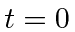when the two coordinate systems are aligned. The forces due to the rotation do not depend on time so this is still general. It will be best to work with the transformation from the rotating (primed) frame to the inertial frame.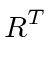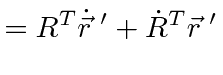This last equation is an important one. It actually holds for the time derivative of any vector, not just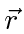. So far it is only true when the coordinate axes coincide like atbut, since it is true for any vector, we can apply it to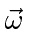.So there is no additional term in the time derivative of.is also unaffected by the rotation and assumed to be constant so we have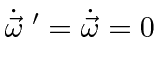and we have for the general vector(at)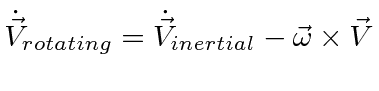whereis the angular velocity vector of the rotating coordinate system in the fixed system.

To get the acceleration (and forces), take the second time derivative.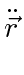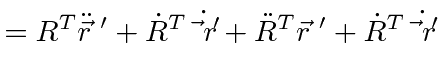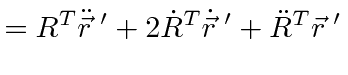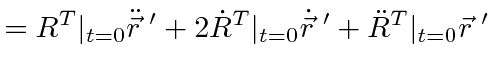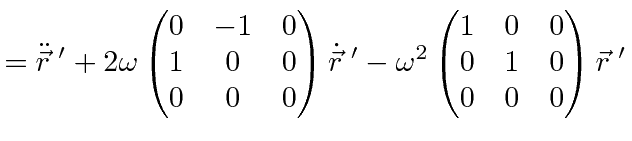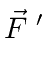This gives us the actual force plus the effective force in the rotating system.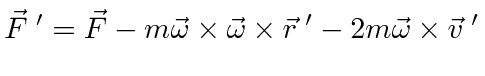The three terms are the actual force, the centrifugal force, and the Coriolis force. The centrifugal force is in the plane of rotation and parallel to. The Coriolis force is perpendicular to both the velocity and.

Now lets go back to the first experiment. First we can compute the velocity in the rotating frame at. (The coordinate axes correspond at.)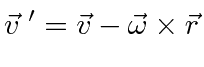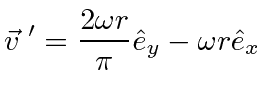This is quite consistent with the path drawn in the diagram.

Now we can compute the effective force in the rotating frame.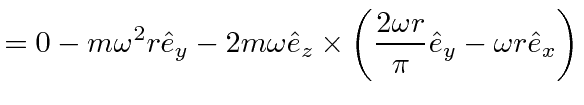This also agrees with the direction of acceleration shown in the diagram at. It would take some effort to integrate the equations to get the exact path. That is more easily done by just transforming the path.

This problem is illustrative but not really the usual type of problem where we know the position and velocity in the rotating frame and need to get the effective forces.

Note that a ball flying above the table would have the same behavior since it is only the radius perpendicular tothat acts in the fictitious forces.

A second simple experiment would be to place the puck at radius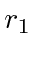on the frictionless rotating table, with no velocity in the inertial frame. The puck would then just remain motionless in the inertial frame and therefore trace out a circle in the rotating frame. Lets check this against our equations.This is exactly the force needed to make the puck go in a circle. The Coriolis force is in the opposite direction of the centrifugal force and is twice as large.

Jim Branson 2012-10-21